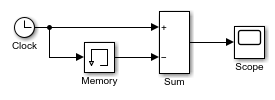# Calculate and Display Simulation Step Size using Memory and Clock Blocks

This example shows how to use the Memory and Clock blocks to calculate and display the step size in a simulation. The Sum block subtracts the time at the previous time step, which the Memory block generates, from the current time, which the Clock block generates.Because Inherit sample time is not selected for the Memory block, the block sample time depends on the type of solver for simulating the model. In this case, the model uses a fixed-step solver. Therefore, the sample time of the Memory block is the solver step size, or 1.

If you replace the Memory block with a Unit Delay block, you get the same results. The Unit Delay block inherits a discrete sample time of 1.